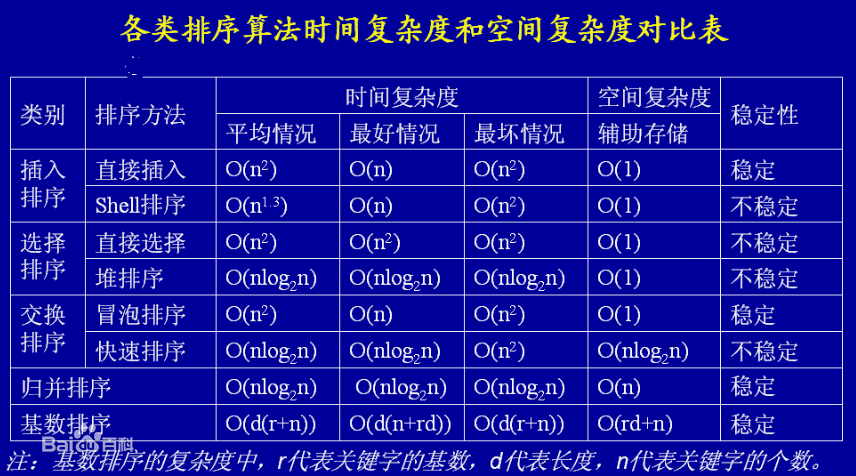# 对数组排序可以说是编程基础中的基础，本文对八种排序方法做简要介绍并用python实现。

• 选择排序

for i in range(len(l)):#意义是第i个位置开始挑第i大（小）的元素
for j in range(i,len(l)):#和其他待排序的元素比较
if l[j]<l[i]:#更大就交换
l[j],l[i]=l[i],l[j]
• 冒泡排序

for i in range(len(l)-1):#下标和i无关，代表的只是第i次排序，最多需要len（l）-1次排序即可
for j in range(len(l)-1):#遍历每一个元素
if l[j]<l[j+1]:#本元素比下一个元素小，就交换
l[j],l[j+1]=l[j+1],l[j]

分析一下其实每次排序都会多一个元素已经确定了位置，不需要再次遍历。

• 插入排序

for i in range(1,len(l)):#意义是第i个元素开始插入i之前的序列（已经有序）
for j in range(i,0,-1):#只要比它之前的元素小就交换
if l[j]<l[j-1]:
l[j],l[j-1]=l[j-1],l[j]
else:
break#直到比前一个元素大

• 归并排序

def gg(l,ll):#合并函数
a,b=0,0
k=[]#用来合并的列表
while a<len(l) and b<len(ll):#两边都非空
if l[a]<ll[b]:
k.append(l[a])
a=a+1
elif l[a]==ll[b]:a=a+1#实现去重
else:
k.append(ll[b])
b=b+1
k=k+l[a:]+ll[b:]#加上剩下的
return k

def kk(p):#分到只剩一个元素就开始合并
if len(p)<=1:
return p
a=kk(p[0:len(p)//2])#不止一个元素就切片
b=kk(p[len(p)//2:])
return gg(a,b)#返回排好序的一部分
l=list(map(int,input().split(" ")))
print(kk(l))
• 快速排序

• 随机化快排

def gg(a,b):
global l
if a>=b:#注意停止条件，我以前没加>卡了半小时
return
x,y=a,b
import random#为了避免遇到基本有序序列退化，随机选点
g=random.randint(a,b)
l[g],l[y]=l[y],l[g]#交换选中元素和末尾元素
while a<b:
if l[a]>l[y]:#比目标元素大
l[a],l[b-1]=l[b-1],l[a]#交换
b=b-1#大于区扩大
#注意：换过以后a不要加，因为新换过来的元素并没有判断过
else:a=a+1#小于区扩大
l[y],l[a]=l[a],l[y]#这时a=b
#现在解释a和b：a的意义是小于区下一个元素
#b是大于区的第一个元素
gg(x,a-1)#左右分别递归
gg(a+1,y)

l=list(map(int,input().split(" ")))
gg(0,len(l)-1)
print(l)

• 堆排序

def down(i,k):#在表l里的第i元素调整，k为边界

#优先队列也是通过这种方式实现的
global l
while 2*i+2<k:#右孩子不越界
lift,right=2*i+1,2*i+2
m=max(l[i],l[lift],l[right])
if m==l[i]:#不需要调
break
if m==l[lift]:#把最大的换上来
l[i],l[lift]=l[lift],l[i]
i=lift#目的节点下标更新
else:#把最大的换上来
l[i],l[right]=l[right],l[i]
i=right#目的节点下标更新
if 2*i+1<k:#判断左孩子
if l[2*i+1]>l[i]:
l[i],l[2*i+1]=l[2*i+1],l[i]

def main():
global l
for j in range(1,len(l)+1):#调大根堆
i=len(l)-j
down(i,len(l))
for i in range(len(l)-1,-1,-1):#排序
l[i],l=l,l[i]#最大和边界交换，剪枝
down(0,i)
print(l)

l=list(map(int,input().split(" ")))
main()


• 桶排序

l=list(map(int,input().split(" ")))
n=max(l)-min(l)
p=*(n+1)#为了省空间
for i in l:
p[i-min(l)]=1#去重排序，做标记即可
for i in range(n):
if p[i]==1:#判断是否出现过
print(i+min(l),end=" ")
• 希尔排序

def shell(arr):
n=len(arr)#初始化步长
h=1
while h<n/3:
h=3*h+1
while h>=1:#判断，退出后就有序了。
for i in range(h,n):
j=i
while j>=h and arr[j]<arr[j-h]:#判断是否交换
arr[j], arr[j-h] = arr[j-h], arr[j]
j-=h
h=h//3#逐渐缩小步长
print arr• 基数排序

（个人认为桶排序名字不恰当，因为桶是先进后出，和稳定的算法正好反了，）

常见的排序算法都是比较排序，非比较排序包括计数排序、桶排序和基数排序，非比较排序对数据有要求，因为数据本身包含了定位特征，所有才能不通过比较来确定元素的位置。

比较排序的时间复杂度通常为O(n2)或者O(nlogn)，比较排序的时间复杂度下界就是O(nlogn)，而非比较排序的时间复杂度可以达到O(n)，但是都需要额外的空间开销。

• 若n较小（数据规模较小），插入排序或选择排序较好
• 若数据初始状态基本有序（正序），插入、冒泡或随机快速排序为宜
• 若n较大，则采用时间复杂度为O(nlogn)的排序方法：快速排序或堆排序
• 快速排序是目前基于比较的排序中被认为是最好的方法，当待排序的关键字是随机分布时，快速排序的平均时间最短；
• 堆排序所需的辅助空间少于快速排序，并且不会出现快速排序可能出现的最坏情况。这两种排序都是不稳定的。

10-297607-13288
05-28374
03-13575
11-1418
09-2874
03-2069
07-20202
10-22153
11-213936
06-13307
08-27262
11-082317
03-05
08-18601
03-0792
©️2020 CSDN 皮肤主题: 书香水墨 设计师:CSDN官方博客